Electrochemistry (2011 VCE)-galvanic cells 1) Two galvanic cells were constructed under standard conditions in an experiment to determine the relative position in the electrochemical series of the standard electrode potential, Eo, for the following reactions. Both cells generate a potential difference.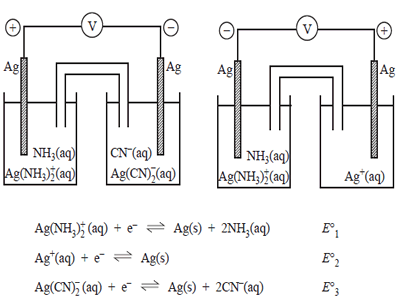The values of the electrode potentials in order from highest to lowest would be. a) Eo1, Eo2 , Eo3 b) Eo1, Eo3 , Eo2 c) Eo2, Eo1, Eo3 d) Eo3, Eo2 , Eo1 Solution. 2) The following galvanic cell was set up under standard conditions..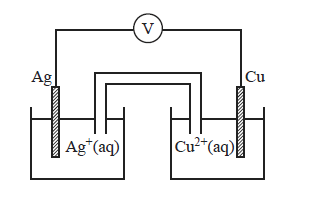The overall equation for the reaction occurring in this galvanic cell is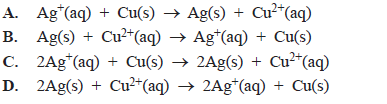Solution3) The expected maximum voltage produced by this cell under standard conditions is a) 0.46 V b) 1.14 V c) 1.26 V d) 1.94 V Solution4) The lead-acid battery is made up of a series of secondary cells in which the following half-reactions are utilised.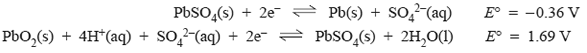i) When the battery is discharging the a) H+ concentration decreases resulting in a higher pH. b) H+ concentration increases resulting in a higher pH. c) H+ concentration decreases resulting in a lower pH. d) H+ concentration increases resulting in a lower pH. Solutionii) When the lead-acid battery is recharging the energy transformation occurring is A chemical => electrical + heat B kinetic => chemical + electrical + heat c) electrical => chemical + heat d) electrical => light + kinetic + heat Solutioniii) The reaction which occurs at the anode when the battery is recharging is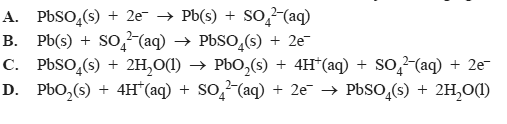Solution iv) When recharging the lead-acid battery the positive terminal of the power supply should be connected to the A. positive terminal of the battery where oxidation will occur. B. positive terminal of the battery where reduction will occur. C. negative terminal of the battery where oxidation will occur. C. negative terminal of the battery where reduction will occur. Solutionv) If we compare a galvanic cell with an electrolytic cell it is true to state that A. in a galvanic cell reduction occurs at the negative electrode B. in both cells the anode is positive and the cathode is negative. C. in an electrolytic cell oxidation occurs at the cathode D. in both cells reduction occurs at the cathode. Solution